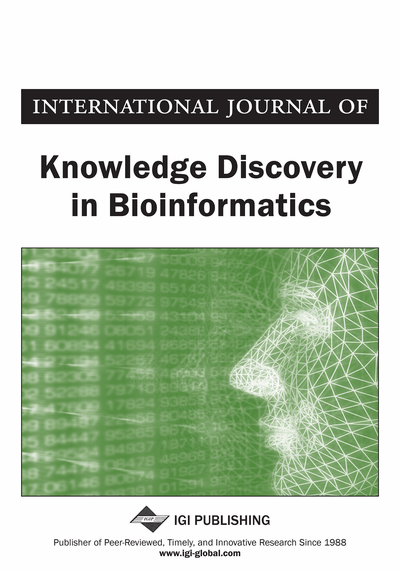# Discriminative Subgraph Mining for Protein Classification

Ning Jin (University of North Carolina, Chapel Hill, USA), Calvin Young (University of North Carolina, Chapel Hill, USA) and Wei Wang (University of North Carolina, Chapel Hill, USA)
DOI: 10.4018/jkdb.2010070103

## Abstract

Protein classification can be performed by representing 3-D protein structures by graphs and then classifying the corresponding graphs. One effective way to classify such graphs is to use frequent subgraph patterns as features; however, the effectiveness of using subgraph patterns in graph classification is often hampered by the large search space of subgraph patterns. In this paper, the authors present two efficient discriminative subgraph mining algorithms: COM and GAIA. These algorithms directly search for discriminative subgraph patterns rather than frequent subgraph patterns which can be used to generate classification rules. Experimental results show that COM and GAIA can achieve high classification accuracy and runtime efficiency. Additionally, they find substructures that are very close to the proteins’ actual active sites.
Article Preview
Top

## Introduction

3-D protein structures can be represented as graphs by replacing each amino acid with a node labeled with the amino acid type and connecting two nodes with an edge labeled with the distance between the two corresponding amino acids if the distance is not too long. Such graph representations preserve important structural information of the proteins and thus a classification of these graphs is in effect a protein classification based on their structures. Performing graph classification by hand is computationally intractable because of the complexity of graphs and the rapidly increasing amount of structural data, so much attention has been devoted to developing graph classification methods.

One solution to graph classification is to use frequent subgraph patterns as graph features and represents each graph as a vector of features. Thus, the problem of graph classification converts to classification of high dimensional data points and many existing generic classification algorithms can be applied. However, one of the major drawbacks of this approach is that when the frequency threshold is low, the number of features may be so large that no existing frequent subgraph mining algorithm can enumerate them with a reasonable amount of computational resource, which is due to the fact that the number of subgraphs is exponential to the number of nodes and edges in graphs. Using high frequency threshold can lead to significant reduction in the number of frequent subgraph patterns, but the discriminative subgraph patterns with lower frequencies than the threshold will be omitted.

To overcome this problem, many approaches have been proposed to mine only discriminative subgraph patterns as features instead of frequent subgraph patterns because only the discriminative subgraph patterns are of use in generating graph classifiers and the number of discriminative subgraph patterns is considerably less than the number of frequent subgraph patterns. SubdueCL (Gonzalez et al., 2002) uses beam search to look for the most discriminative subgraph pattern iteratively until each positive graph can be covered by at least one subgraph pattern. However, its efficiency is low for it calculates subgraph frequency by computing subgraph-isomorphism, which is an NP-complete problem. In (Kudo et al., 2004), the authors integrate the AdaBoost algorithm with gSpan (Yan & Han, 2002), an efficient frequent subgraph mining algorithm. They adapt gSpan to implement a branch-and-bound search to find the subgraph feature with the highest gain. Leap (Yan et al., 2008) is another algorithm developed to mine the most discriminative subgraph pattern. Leap uses two novel mining techniques, structural proximity pruning and frequency-descending mining, to excel the aforementioned branch-and-bound search algorithm. The structural proximity pruning takes into account the fact that subgraph patterns that share a large subgraph have similar discrimination power and uses it to calculate a tight upper-bound of their discrimination scores. The frequency-descending mining takes advantage of the observation that subgraphs with higher frequency are more likely to be discriminative and thus may reach the optimal solution faster. Another algorithm, gPLS (Saigo et al., 2008), uses subgraph pattern mining to find features for partial least squares regression so that the mining process only searches for patterns that can improve the accuracy of the resulting classifier. The algorithms mentioned above are not efficient because they find one discriminative subgraph pattern at a time and must be invoked repeatedly to find enough discriminative subgraph patterns to generate graph classifiers, thereby limiting their ability to process large datasets.

## Complete Article List

Search this Journal:
Reset
Open Access Articles
Volume 8: 2 Issues (2018)
Volume 7: 2 Issues (2017)
Volume 6: 2 Issues (2016)
Volume 5: 2 Issues (2015)
Volume 4: 2 Issues (2014)
Volume 3: 4 Issues (2012)
Volume 2: 4 Issues (2011)
Volume 1: 4 Issues (2010)
View Complete Journal Contents Listing